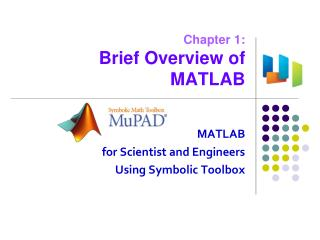DownloadDownload PresentationChapter 1: Brief Overview of MATLAB

# Chapter 1: Brief Overview of MATLAB

Download Presentation## Chapter 1: Brief Overview of MATLAB

- - - - - - - - - - - - - - - - - - - - - - - - - - - E N D - - - - - - - - - - - - - - - - - - - - - - - - - - -
##### Presentation Transcript

1. Chapter 1:Brief Overview of MATLAB MATLAB for Scientist and Engineers Using Symbolic Toolbox

2. You are going to • Exposeyourself to the history of MATLAB, • See what MATLAB provides, • Look at a symbolic math example.

3. Old History of MATLAB • 1967: "Computer solution of linear algebraic equations", Forsythe and Moler • 1976: "Matrix Eigensystem Routines, EISPACK Guide" in FORTRAN • 1976~9: "LINPACK" in FORTRAN • 1977~: "MATLAB Environment", Cleve Moler • 1971: "Handbook for automatic computations" in ALGOL, • J. H. Wilkinson et. al. Cleve Moler (1939~) J.H.Wilkinson, UK (1919~1986) • 1979: "Numerical analysis" lecture at Stanford, • met with Jack Little, then an engineering student • 1984: MathWorks founded by Jack and Moler Jack Little

4. Further Digging Resources • Video: The Origins of MATLAB at MathWorks.com • http://www.mathworks.com/company/aboutus/founders/clevemoler.html • Meet MrMatlab at Scientific Computing World • http://www.scientific-computing.com/features/feature.php?feature_id=15 • Cleve Moler at Wikipedia • http://en.wikipedia.org/wiki/Cleve_Moler • BLAS at Netlib.org • http://www.netlib.org/blas/ • Maple at Wikipedia and Maplesoft.com • http://en.wikipedia.org/wiki/Maple_(software) • http://www.maplesoft.com/index1.aspx

5. MATLAB? Matrix Laboratory Matrix Arithmetic, Eigen Analysis, ... >> c=a*b c = 32 77 80 54 62 105 64 41 100 >> d=a/b d = 2.3934 2.0164 -2.1639 0.1475 0.1311 0.6885 5.0820 1.2951 -2.9508 >> b=floor(10*rand(3)) b = 1 5 3 6 1 8 3 6 8 >> a=magic(3) a = 8 1 6 3 5 7 4 9 2

6. Graphics & Visualization

7. Graphical User Interface fifteen fdatool Try them for yourself! xpbombs

8. Toolboxes

9. SIMULINK • Model-based design environment Ref: Help – Video and Image Processing Blockset – Demos – Motion Detection

10. They Use MATLAB for … • Math and computation • Algorithm development • Data acquisition • Modeling, simulation, and prototyping • Data analysis, exploration, and visualization • Scientific and engineering graphics • Application development, including graphical user interface building Ref: Help – MATLAB – Getting Started – Introduction – Product Overview

11. The MATLAB System The MATLAB system consists of these main parts: • Desktop Tools and Development Environment • Mathematical Function Library • The Language • Graphics • External Interfaces; API + • Toolboxes: MATLAB function packages • Simulink: Model-based design • Blocksets: Simulink model packages Ref: Help – MATLAB – Getting Started – Introduction – Product Overview

12. What is Symbolic Computing • Position of an oscillating mass: • Velocity? General Solution View underlying mathematics Ref: Webinar – Symbolic Computing Tools for Academia

13. Symbolic Math Usage in Academia Ref: Webinar – Symbolic Computing Tools for Academia

14. Working w/t Symbolic Math Toolbox From Notebook Interface • Conveniently manage & document symbolic computations • Math notation, embedded text, graphics • Access complete MuPAD language • 15+libraries of symbolic math functions From MATLAB • Perform symbolic computations using familiar MATLAB syntax Sharing Ref: Webinar – Symbolic Computing Tools for Academia

15. Demo: Mass-Spring-Damp System Dynamic Equation Initial Conditions Symbolic Math Solution Ref: Webinar – Symbolic Computing Tools for Academia mass_spring_damp_system.mn

16. Symbolic Math Toolbox Libraries • Calculus • Differentiation • Integrals • Jacobian • Taylor series • Limits • Solving Equations • Algebraic Equations • Differential Equations • Transforms • Fourier transform • Laplace transform • Z-transform • Simplification • Polynomial Expansion • Substitution • Linear Algebra • Operations • Eigenvalues • Special Functions • Bernoulli, Bessel, Beta, … • Fresnel sine/cosine integral, Gamma • Variable Precision Arithmetic • Plotting • 2-D • 3-D contour, surface, mesh • Animations Ref: Webinar – Symbolic Computing Tools for Academia